# Sorting Counting Pigeon Hole Bucket Sort Radix Sort

• Slides: 17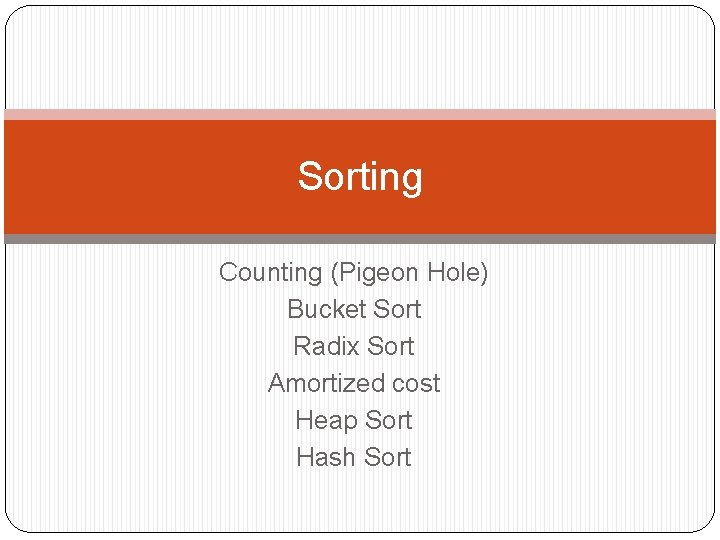Sorting Counting (Pigeon Hole) Bucket Sort Radix Sort Amortized cost Heap Sort Hash SortCounting Sort 5 pigeonhole(A) (a. k. a. counting sort) // it is known that all values in A are integers between 1 and 10, 000 for k 1 to 10, 000 // u is an array that contains the counts of each integer in A u[k] 0 // set the counts to zero for i 1 to n do // go through A k A[i] // read each integer in A u[k] + 1 // add 1 to the count of that integer i 0 // prepare to walk through the A array for k 1 to 10, 000 // go through the counts array while u[k] 0 // for each time a particular integer was in the array i i + 1 A[I] k // write that integer in the A array u[k] – 1 // decrement the counts array entry for that array. The running time is O(10, 000 + n)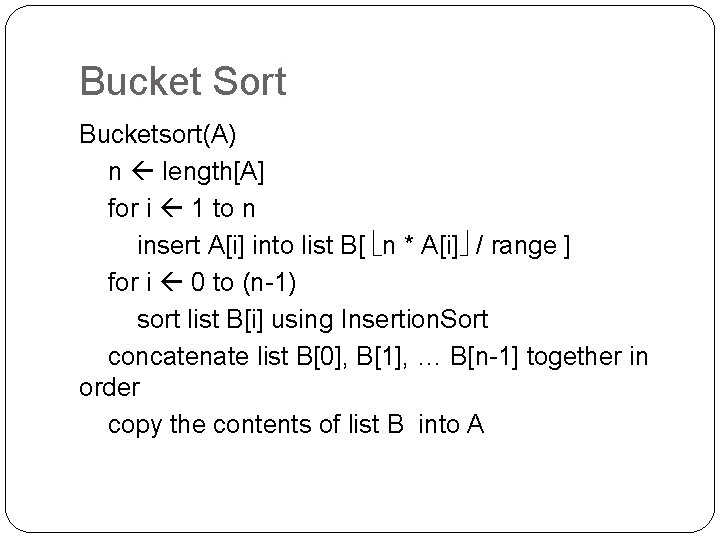Bucket Sort Bucketsort(A) n length[A] for i 1 to n insert A[i] into list B[ n * A[i] / range ] for i 0 to (n-1) sort list B[i] using Insertion. Sort concatenate list B, B, … B[n-1] together in order copy the contents of list B into A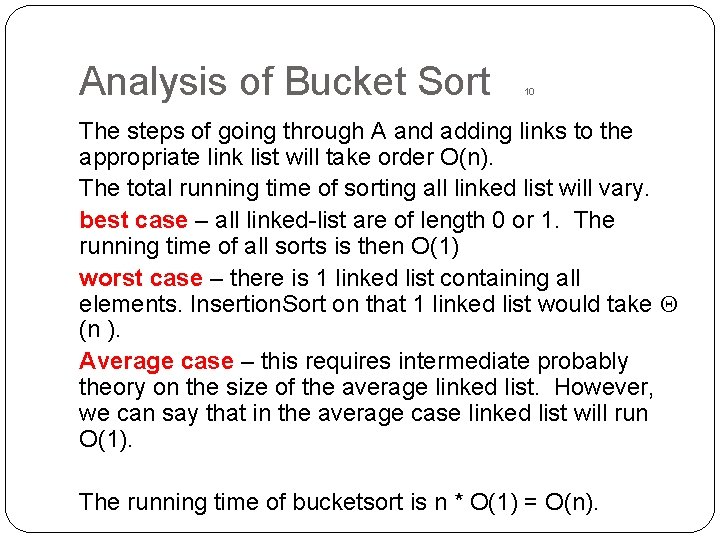Analysis of Bucket Sort 10 The steps of going through A and adding links to the appropriate link list will take order O(n). The total running time of sorting all linked list will vary. best case – all linked-list are of length 0 or 1. The running time of all sorts is then O(1) worst case – there is 1 linked list containing all elements. Insertion. Sort on that 1 linked list would take (n ). Average case – this requires intermediate probably theory on the size of the average linked list. However, we can say that in the average case linked list will run O(1). The running time of bucketsort is n * O(1) = O(n).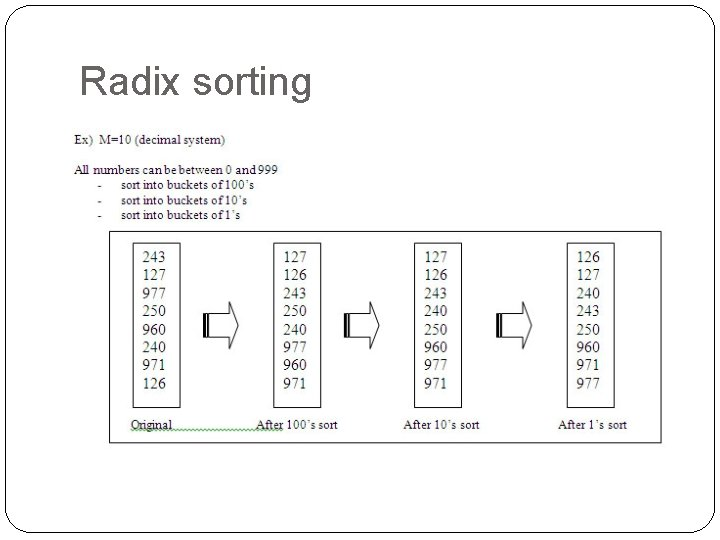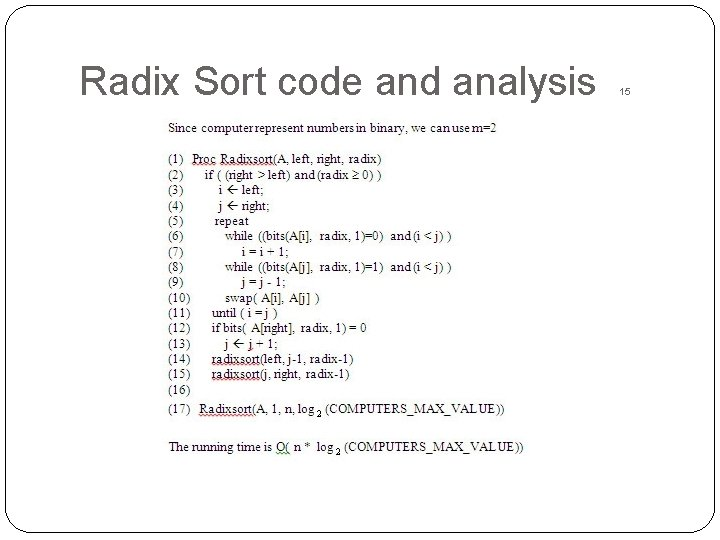Radix Sort code and analysis 15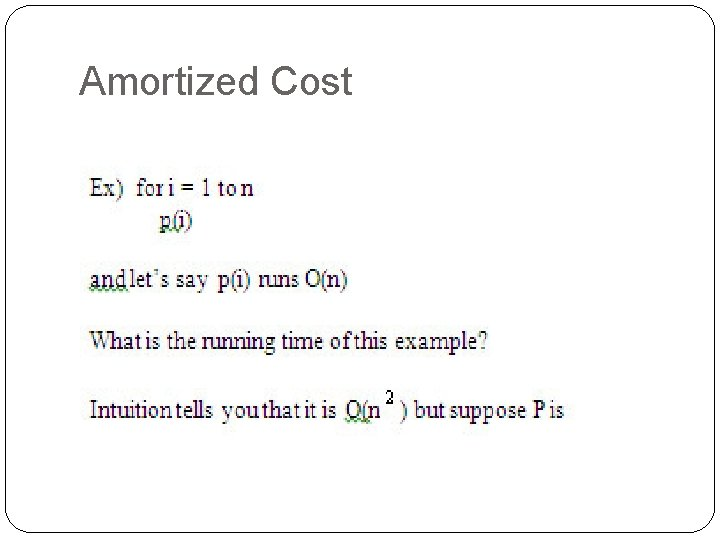Amortized Cost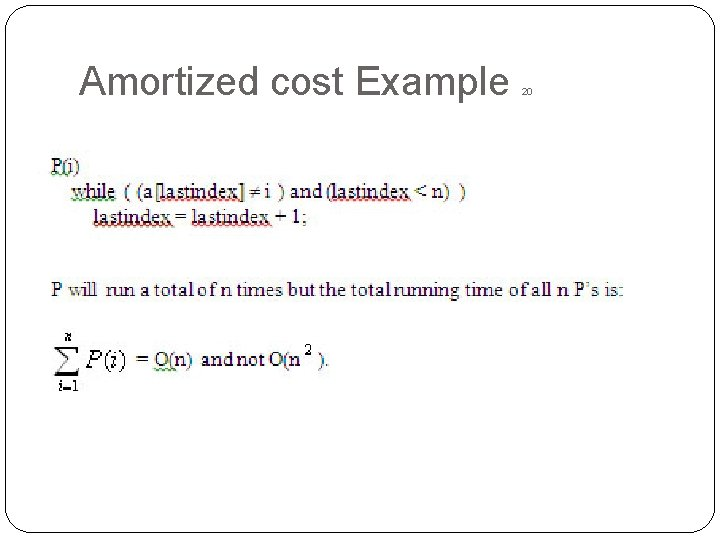Amortized cost Example 20Heap Sort There are 3 procedures. heapify takes an array A and an index i. It traverses down the tree binary heap and insert the passed in index value in the array into it’s correct location in the heap. buildheap which will call heapify to insert all elements into the heap. Calling build heap on a arbitrary array will turn it into a binary heapsort will take an arbitrary array, call buildheap to turn the array into a binary heap. Then will pop the top element out of the heap into the answer array and between each pop, will re-heapify the array so to maintain the heap property.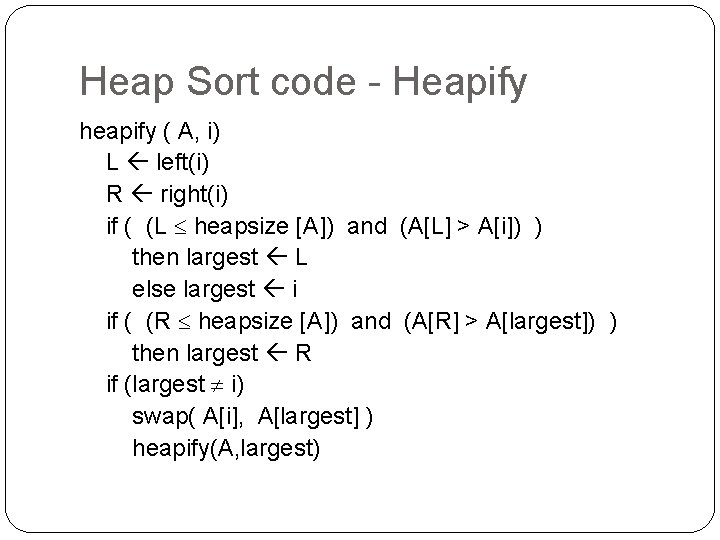Heap Sort code - Heapify heapify ( A, i) L left(i) R right(i) if ( (L heapsize [A]) and (A[L] > A[i]) ) then largest L else largest i if ( (R heapsize [A]) and (A[R] > A[largest]) ) then largest R if (largest i) swap( A[i], A[largest] ) heapify(A, largest)Heap Sort – buildheap/heapsort buildheap(A) heapsize length(A); for i length[A] / 2 downto 1 heapify(A, i); heapsort(A) buildheap(A) for i length[A] downto 2 swap(A, A[i] ) heapsize = heapsize – 1 heapify( A, 1)Heap Sort – Analysis 30 Heapify runs O(lg n). Buildheap calls heapify n/2 times so it runs O(n lg n) Heapsort calls buildheap once which run O(n lg n) and then calls (swap, a subtraction operating and heapify) n/2 times which runs: O(n/2 * (O(1) + O(lg n) ) which is O(n lg n). Since heapsort runs 2 steps, each running O(n lg n), the overall algorithm runs O(n lg n).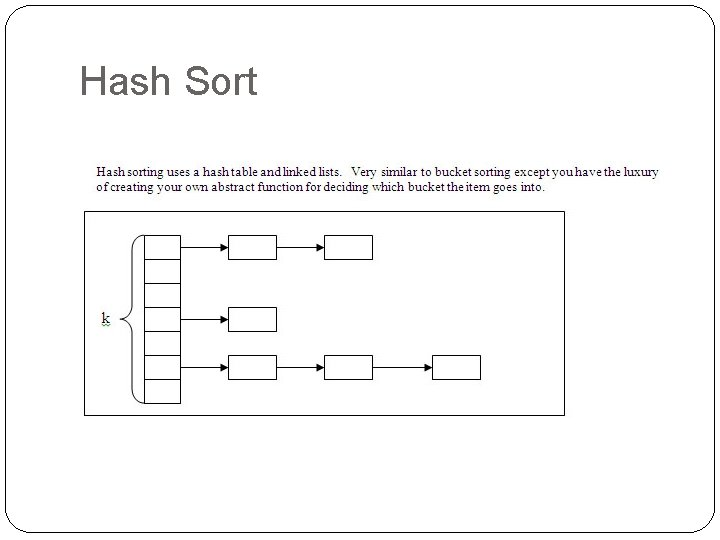Hash SortHash Sort for i i to n j hashfunction ( A[i] ) // get a linklist to add this into add (A[i], linklist(j) ) // insert this value into the sorted linked-list for i 1 to k copy (linklist(i) , A); Running time O(n * O(hashfunction + add) + k * O(copy) If you choose a hash function that runs O(1), then the amortized cost of step 2 is O(k + n) Note: if K << n then step 2 runs O(n)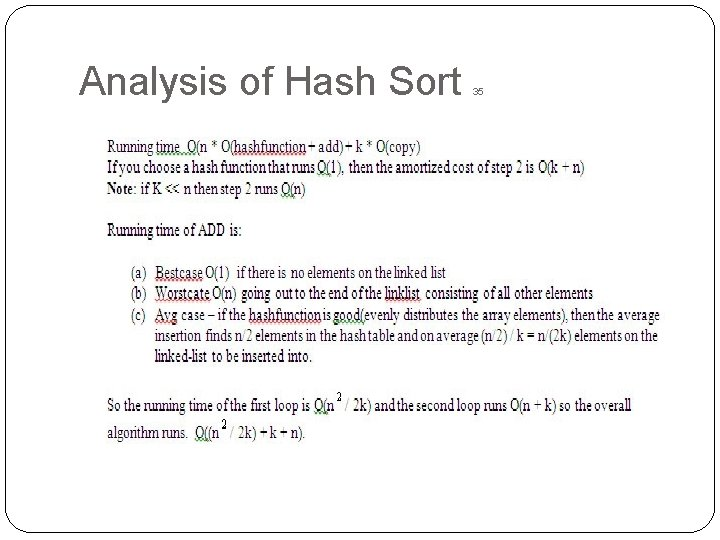Analysis of Hash Sort 35Comparison Sorting is O(n lg n) Some sorting algorithms run O(n lg n) – - Quicksort - Heapsort - Mergesort Some algorithms run linear time - Counting sort - Radix sort - Hashsort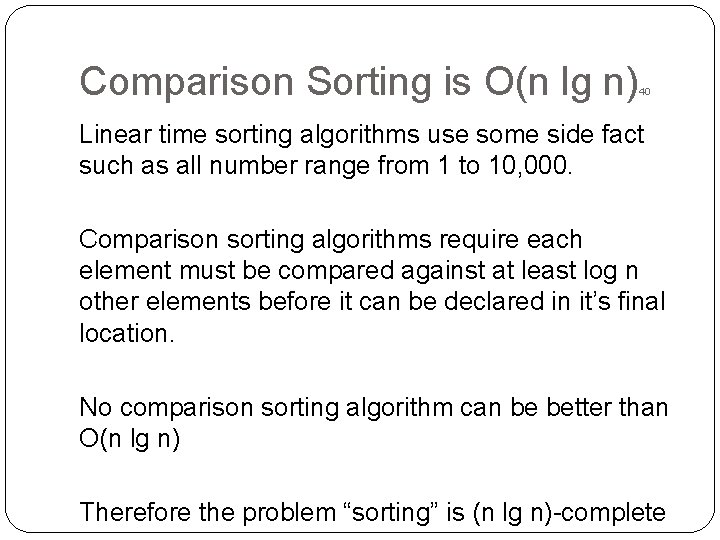Comparison Sorting is O(n lg n) 40 Linear time sorting algorithms use some side fact such as all number range from 1 to 10, 000. Comparison sorting algorithms require each element must be compared against at least log n other elements before it can be declared in it’s final location. No comparison sorting algorithm can be better than O(n lg n) Therefore the problem “sorting” is (n lg n)-complete